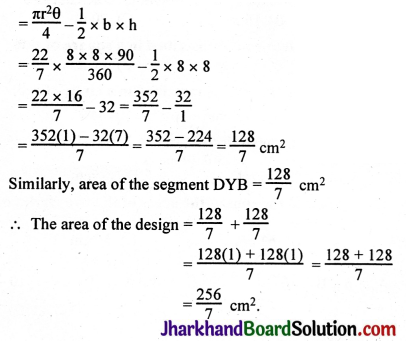# JAC Class 10 Maths Solutions Chapter 12 Areas Related to Circles Ex 12.3

Jharkhand Board JAC Class 10 Maths Solutions Chapter 12 Areas Related to Circles Ex 12.3 Textbook Exercise Questions and Answers.

## JAC Board Class 10 Maths Solutions Chapter 12 Areas Related to Circles Exercise 12.3

Unless stated otherwise, use π = $$\frac{22}{7}$$

Question 1.
Find the area of the shaded region in the figure, if PQ = 24 cm, PR = 7 cm and O is the centre of the circle.Solution:
Given, PQ = 24 cm, PR = 7 cm.
We know any triangle drawn from diameter RQ to the circle is 90°.
Here, ∠RPQ = 90°
In right ΔRPQ, RQ2 = PR2 + PQ2 (By Pythagoras theorem)
RQ2 = 72 + 242
RQ2 = 49 + 576
RQ2 = 625
RQ = 25 cm
∴ Area of ΔRPQ = $$\frac{1}{2}$$ × RP × PQ
= $$\frac{1}{2}$$ × 7 × 24 = 84 cm2
∴ Area of semi-circle = $$\frac{\pi r^2}{2}=\frac{22}{7 \times 2}\left(\frac{25}{2}\right)^2$$ (∵ r = $$\frac{\mathrm{PQ}}{2}=\frac{25}{2}$$ cm)
= $$\frac{11 \times 625}{28}=\frac{6875}{28} \mathrm{~cm}^2$$
∴ Area of the shaded region = Area of the semi-circle – Area of right ΔRPQ
= $$\frac{6875}{28}-84$$
= $$\frac{6875-2352}{28}=\frac{4523}{28}$$ cm2.Question 2.
Find the area of the shaded region in the figure, if radii of the two concentric circles with centre O are 7 cm and 14 cm respectively and ∠AOC = 40°.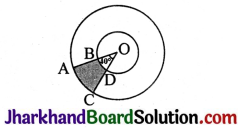Solution:
R – radius of the bigger circle, r – radius of the smaller circle.
Area of the shaded portion = Area of sector OAC – Area of sector OBD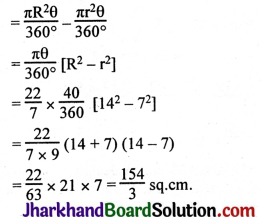Question 3.
Find the area of the shaded region in the figure, if ABCD is a square of side 14 cm and APD and BPC are semicircles.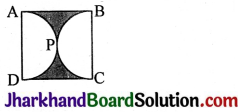Solution:
Area of the shaded portion = Area of the square – 2 × Area of one semicircle
= (14 × 14) – 2 × $$\frac{\pi r^2}{2}$$ [∵ r = 7]
= 14 × 14 – 2 × $$\frac{22}{7} \times \frac{7 \times 7}{2}$$
= 196 – 154 = 42 cm2.

Question 4.
Find the area of the shaded region in the figure, where a circular are of radius 6 cm has been drawn with vertex O of an equilateral triangle OAB of side 12 cm as centre.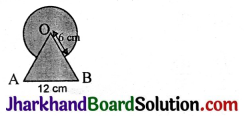Solution:
Area of the shaded portion = Area of the circle of radius 6 cm + Area of equilateral ΔABC – Area of the sector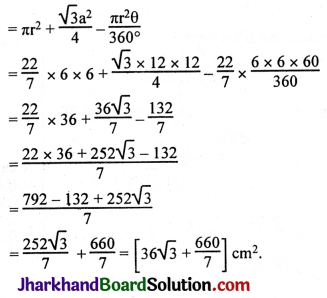Question 5.
From each corner of a square of side 4 cm a quadrant of a circle of radius 1 cm is cut and also a circle of diameter 2 cm is cut as shown in the figure. Find the area of the remaining portion of the square.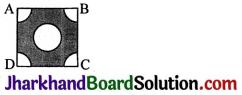Solution:
Area of the shaded portion = Area of the square – Area of the 4 quadrants – Area of the circle
= (4 × 4) – 4 × area of one quadrant – area of the circle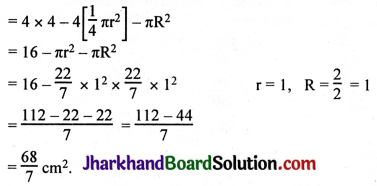Question 6.
In a circular table cover of radius 32 cm, a design is formed leaving an equilateral triangle ABC in the middle as shown in the figure. Find the area of the design.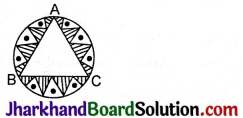Solution:
Area of the design (shaded region) = Area of the circle – Area of ΔABC
Area of equilateral triangle ABC = $$\frac{\sqrt{3} a^2}{4}$$
In ΔABC, AL ⊥ BC.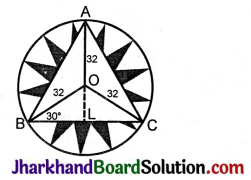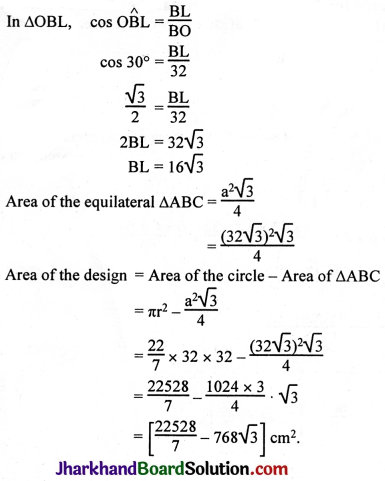Alternative Method: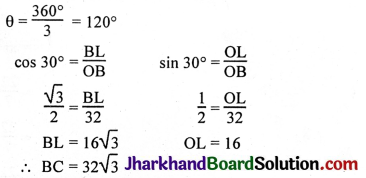= 3[Area of segments]
= 3[Area of sector – Area of ΔOBC]
= 3[$$\pi r^2 \frac{\theta}{360^{\circ}}-\frac{1}{2}$$ × BC × OL]

Question 7.
In the figure, ABCD is a square of side 14 cm. With centres A, B, C and D, four circles are drawn such that each circle touches externally two of the remaining three circles. Find the area of the shaded region.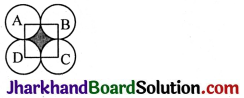Solution:
Area of the shaded region = Area of the square – Area of the 4 quadrants
= Area of the square – 4 × area of one quadrant
= (14)2 – 4 × $$\frac{1}{4}$$πr2
= (14)2 – 4 × $$\frac{1}{4} \times \frac{22}{7}$$ × 7 × 7
= 196 – 154
= 42 cm2.Question 8.
The figure depicts a racing track whose left and right ends are semicircular.
The distance between the two inner parallel line segments is 60 m and they are each 106 m. long. If the track is 10 m wide, find:
(i) the distance around the track along its inner edge.
(ii) the area of the track.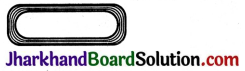Solution:
i) Distance around the inner track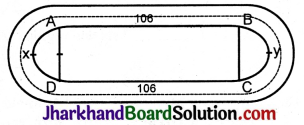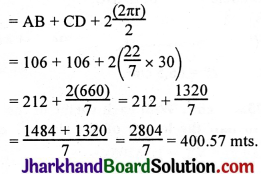ii) Area of the track: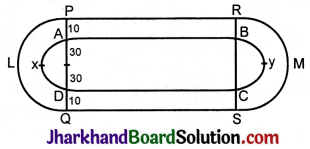Area of the track = l × b + l × b + 2$$\left[\frac{\pi \mathrm{R}^2}{2}-\frac{\pi \mathrm{r}^2}{2}\right]$$ r = 30 mts, R = (30 + 10) = 40 mts.
= 106 × 10 + 106 × 10 + 2 × $$\frac{\pi}{2}$$(R2 – r2)
= 1060 + 1060 + $$\frac{22}{7}$$[402 – 302]
= 2120 + $$\frac{22}{7}$$[1600 – 900]
= 2120 + $$\frac{22}{7}$$
= 2120 + 2200
= 4320 m2.

Question 9.
In the figure, AB and CD are two diameters of a circle (with centre O) perpendicular to each other and OD is the diameter of the smaller circle. If OA = 7 cm, find the area of the shaded region.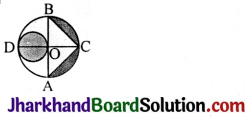Solution:
Given,
OA = 7 cm
∴ OD = 7 cm
Now, area of smaller circle whose diameter (OD) = 7 cm is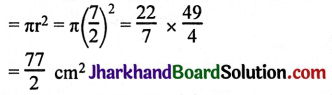Now,
Area of ΔABC = $$\frac{1}{2}$$ × AB × OC
= $$\frac{1}{2}$$ × 2 × OA × OC
= $$\frac{1}{2}$$ × 14 × 7 (∵ OA = OC)
= 49 cm2.
Area of semi-circle ABCA = $$\frac{\pi r^2}{2}=\frac{22}{7 \times 2}(7)^2$$
= 77 cm2
∴ Area of segments BC and AC = Area of semi-circle – Area of AABC
= 77 – 49 = 28 cm2
∴ Area of total shaded region = Area of small circle + Area of segments BC and AC
= $$\frac{77}{2}$$ + 28
= 38.5 + 28
= 66.5 cm2.Question 10.
The area of an equilateral triangle ABC is 17320.5 cm2. With each vertex of the triangle as centre, a circle is drawn with radius equal to half the length of the side of the triangle. Find the area of the shaded region. (Use π = 3.14 and $$\sqrt{3}$$ = 1.73205).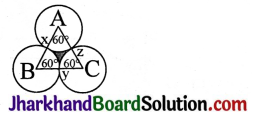Solution:
Area of the shaded portion = Area of equilateral ΔABC – Area of sector Axy – Area of sector Bxz – Area of sector Cyz.
π = 3.14, θ = 60°, r = ?, r = $$\frac{a}{2}$$
Area of the shaded portion = Area of the equilateral Δ – 3 × Area of one sector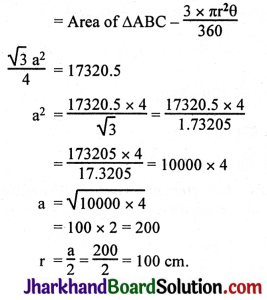Area of the shaded region = Area of ΔABC – $$\frac{3 \times \pi r^2 \theta}{360}$$
= 17320.5 – $$\frac{3 \times 3.14 \times 100 \times 100 \times 60}{360}$$
= 17320.5 – 1.57 × 10000
= 17320.5 – 15700.0
= 1620.5 sq.cm.

Question 11.
On a square handkerchief, nine circular designs each of radius 7 cm are made. Find the area of the remaining portion of the handkerchief.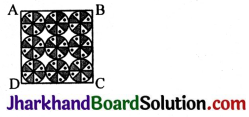Solution:
Number of circular designs = 9
Radius of the circular design = 7 cm
There are three circles in one side of the square handkerchief.
∴ Side of the square = 3 × diameter of circle = 3 × 14 = 42 cm
Area of the square = 42 × 42 cm2 = 1764 cm2
Area of the circle = πr2 = $$\frac{22}{7}$$ × 7 × 7 = 154 cm2
Total area of the design = 9 × 154 = 1386 cm2
Area of the remaining portion of the handkerchief = Area of the square – Total area of the design
= 1764 – 1386
= 378 cm2.

Question 12.
In the figure, OACB is a quadrant of a circle with centre O and radius 3.5 cm. If OD = 2 cm find the area of the (i) quadrant OACB, (ii) shaded region.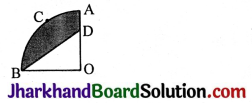Solution: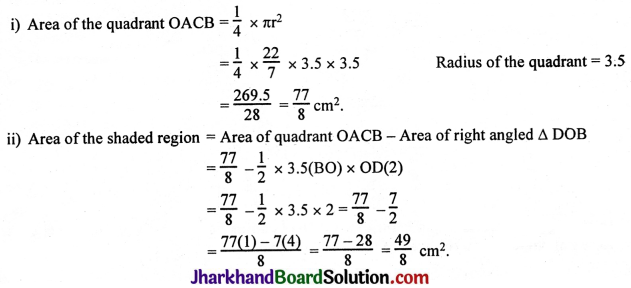Question 13.
In the figure, a square OABC is inscribed in a quadrant OPBQ. If OA = 20 cm, find the area of the shaded region. (Use π = 3.14)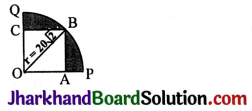Solution:
OB2 = OA2 + AB2
= 202 + 202
= 400 + 400
= 800
OB2 = 400 × 2
OB = $$\sqrt{400 \times 2}$$ = 20$$\sqrt{2}$$
Area of the shaded region = Area of the quadrant OPBQ – Area of the square OABC
= $$\frac{1}{4}$$ πr2 – (OA)2
= $$\frac{1}{4}$$ × 3.14 × 20$$\sqrt{2}$$ × 20$$\sqrt{2}$$ – (20)2
= $$\frac{1}{4}$$ × 3.14 × 400 × $$\sqrt{4}$$ – 400
= $$\frac{1}{4}$$ × 3.14 × 400 × 2 – 400
= 100 × 2 × 3.14 – 400
= 100 × 2(3.14 – 2)
= 200 × 1.14
= 228 sq.cm.Question 14.
AB and CD are respectively arcs of two concentric circles of radii 21 cm and 7 cm and centre O. If ∠AOB = 30°, find the area of the shaded region.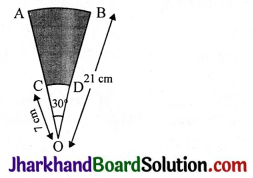Solution:
R = 21, r = 7, θ = 30°, π = $$\frac{22}{7}$$
Area of the shaded region = Area of sector OAB – Area of sector OCD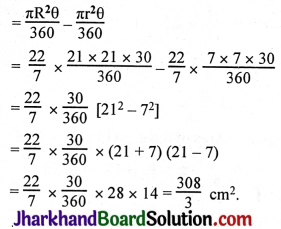Question 15.
In the figure, ABC is a quadrant of a circle of radius 14 cm and a semicircle is drawn with BC as diameter. Find the area of the shaded region.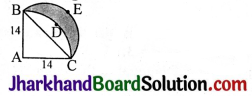Solution:
BC2 = 142 + 142
BC2 = 196 + 196 = 392
BC = $$\sqrt{392}$$ = $$\sqrt{196 \times 2}$$ = 14$$\sqrt{2}$$ = d
r = $$\frac{14 \sqrt{2}}{2}=7 \sqrt{2}$$
Radius of the sector = 14.
Area of the shaded region Area of the semicircle BEC – Area of the segment BDCB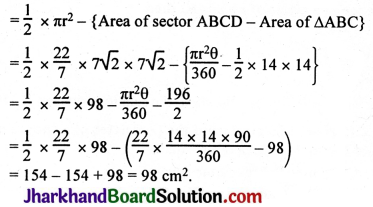Question 16.
Calculate the area of the designed region in the figure common between the two quadrants of circles of radius 8 cm each.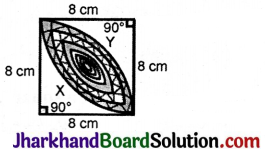Solution:
Area of the design = Area of sector DXB + Area of ΔDCB
Area of the segment DXB = Area of the sector DXBC – Area of ΔDCB